# Equivocal Function Transformations

The last two posts were about transformations of functions (shift, stretch, reflect) and their effect on a graph, first individually and then in combination. The next thing to look at will be how to determine the transformations when you are given a graph; but before we take that challenge in general, we need to see how transformations can interact with particular base functions, resulting in graphs that can be attained in more than one way. This can make it hard to decide what to call it; or it can actually make things easier, by letting us choose the easier of two ways.

## Square roots: compressed or stretched?

We’ll first look at this question from 2009:

Square Root Functions and Transformations

How can we tell by looking at a graph if a square root function is being horizontally compressed or vertically stretched?

For example, y = 2 sqrt(x) is being vertically stretched when graphed, but y = sqrt(2x) is being horizontally compressed.

I'm confused because they both graphically look like they are being horizontally compressed or vertically stretched.  However, my textbook makes a distinction for both cases.  Ultimately, I would like to know how to tell the difference by merely looking at a graph.

Jon has been given two functions to graph, and astutely observed that the results of a vertical stretch and a horizontal compression look essentially alike. What will he do when he is given a graph and asked to determine the function?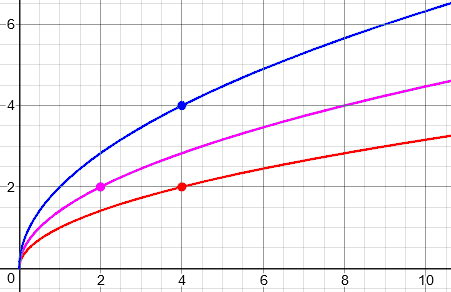The red graph is $$f(x)=\sqrt{x}$$, and the red point is (4, 2). A vertical stretch produces the blue graph, $$g(x)=2\sqrt{x}$$, with the point, (4, 4) showing the doubling of y. A horizontal compression by the same factor produces the purple graph, $$h(x)=\sqrt{2x}$$, with the point, (2, 2) showing the halving of x. There is no difference between the effects of the two; in fact, as Doctor Ali pointed out, we could have obtained the exact same graph two ways:

Hi Jon!

Thanks for writing to Dr. Math.  You asked a very nice question.

The answer is, we can't tell!  Note that in the example you gave, we can write

y = Sqrt(2x)

as

y = Sqrt(2) Sqrt(x) ~= 1.4142 Sqrt(x)

So, if we just have the graph, is it horizontally compressed by 1/2 or vertically stretched by 1.4142?  We can't tell.

Does that make sense?  Please write back if you still have any difficulties.

That is, our purple graph above could have been obtained by vertical stretching, as shown here by the dot at $$(4, 2\sqrt{2})$$: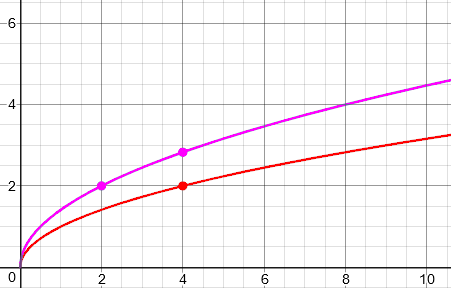It may be important to point out that not all functions behave like the square root.  In general, horizontal compression and vertical stretch are NOT equivalent.  (Try doing the same thing with a general linear function, y=ax+b.)  In the case of the square root, it happens that we can describe the same transformation either way, making it an arbitrary choice, but that is not usually true.

So the bottom line for Jon is that it doesn’t matter: We could have described the transformation either way, and we would be correct. It is impossible to say how the originator of a given graph obtained it; but either equation is correct because they are equivalent: $$h(x)=\sqrt{2x}=\sqrt{2}\sqrt{x}$$.

This is due to the product property of the square root: $$\sqrt{ax}=\sqrt{a}\sqrt{x}$$. Functions that lack this, or a similar property, will not have this ambiguity.

## Squaring: are they really different transformations?

Another function with a similar property is the square. This led to a different perspective on the issue in a 2014 question:

Shifting Images

When I change from a horizontal compression to a corresponding vertical stretch, why does the image point change?

For example, consider f(x) = x^2.

Applying a horizontal compression of 1/3 and a shift left by 4 units yields y = [3(x + 4)]^2.

The preimage point is (1, 1), and the image is (-3 2/3, 1).

Applying a vertical stretch of 9 and a shift left 4 units yields y = 9(x + 4)^2.

This gives the exact same graph, but now (1, 1) goes to image (-3, 9).

Why? Why does basically the same transformation yield different image points for the same preimage?

Here we are combining a compression and a shift (using the format I recommended last time), then expanding into a form that pulls the 3 outside and squares it, changing the horizontal compression to a vertical stretch. These are the same function, but obtained by two different transformations. Here are the graphs and the points referred to, showing how the point (1, 1) transforms. First, horizontal compression (purple) followed by shift (blue):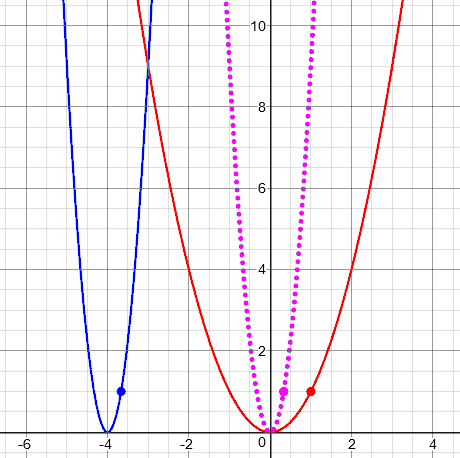Second, vertical stretch (purple) followed by shift (blue):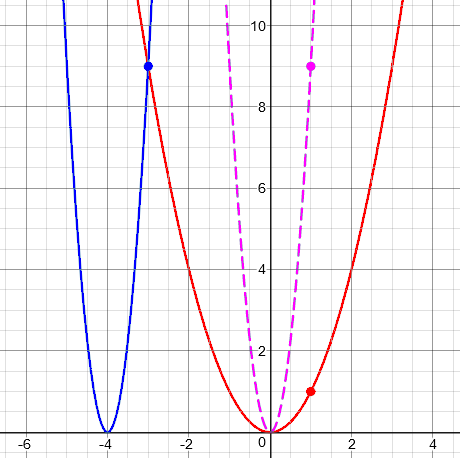Both transformations have the same effect on the graph, but different effects on an individual point.

I replied, initially just pointing this out:

These are not the same transformation. They are two different transformations that transform this particular graph into the same graph.

The point (1, 1), which is on the original graph, is mapped to different points by the two transformations, but both are on the graph of the same transformed function.

So the real question is, why do these two different transformations transform f into the same function?

Rhonda replied with a question like the previous one:

Thank you!

Your answer raises the very question I still have: "Why do these two different transformations transform f into the same function?"

I understand that the order in which transformations are performed can sometimes yield different results, and sometimes yield the same result. What concerns me is vertical and horizontal stretches and compressions.

On some functions, like quadratics, a horizontal compression of 1/3 yields the same result as a vertical stretch of 9. But when applied to a sine function, a horizontal compression of 1/3 does not yield the same result as a vertical stretch of 3.

Is there a "rule" as to when it matches up and when it doesn't?

It's a special property of the function.

In the case of f(x) = x^2,

f(ax) = (ax)^2
= a^2x^2
= a^2f(x)

So a horizontal compression by 1/a is equivalent to a vertical stretch by a^2. This is not true for the sine.

Any function with this sort of property will have pairs of transformations as in your example. Other properties of functions might yield different relationships between transformations; for instance, a horizontal stretch of a log is the same as a vertical translation:

log(ax) = log(a) + log(x)

This last comment points out a property of the logarithm function that makes a horizontal stretch equivalent to a vertical shift! I didn’t expand on that idea, but here is an example:

The function $$log_2(2x)$$ represents a horizontal shrink by a factor of 2, taking (2,1) to (1, 2); but rewriting it as $$log_2(2)+log_2(x)= log_2(x)+1$$ makes it a vertical shift, taking (2, 1) to (2, 2).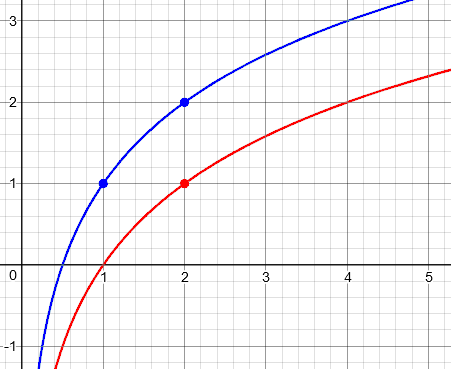Something similar is true of an exponential function.

In each of these cases, it would be easier in practice to do the vertical transformation rather than the horizontal, which tends to be more confusing (as in Rhonda’s example, where she masterfully handled the mix of two horizontal transformations, but it took extra effort, and many students would have done poorly). So these mutable transformations can provide ways to save trouble in graphing or in writing the formula from a graph.

Let’s look at a 2016 question that takes Rhonda’s last question a step further:

Dilation Designation: Why in the Family of Trigonometric Functions Rather than Quadratics?

Why are horizontal dilations usually included in trigonometric functions rather than in the family of quadratic functions or absolute value functions? Is it possible to generate ALL quadratic functions without needing to include horizontal dilations? If so, what is it about trig functions that requires them to have a horizontal dilation in order to create the whole family?

More explicitly, is there a mathematical reason trig function families are often described this way ...

g(x) = a * f(bx + c) + d
= a sin(bx + c) + d

... while quadratic and absolute value function families are usually described with merely this?

g(x) = a * f(x - b)   + c
= a *  (x - b)^2 + c

Is there something about the nature of the functions themselves that makes the b redundant in some families?

Graphing the trig functions leads me to believe that b seems important. Does that have anything to do with the periodic nature of some functions?

It seems like ALL absolute value functions and quadratic functions are possible without the b. But I can't even come close to proving it. I wouldn't even know where to start.

Mark is using the term “dilation” where I have been mostly using the more informal term “stretch or shrink/compress” in line with students’ questions. One reason to prefer his term is that one word covers both directions. Math terms often work this way.

Having seen the previous answers, you may see where we need to go with this. What I like to do, when a student asks “Why can’t I …” or “What don’t I have to …” is to suggest that they try it and see. Here is my reply:

I'll add a horizontal dilation into your general form for a quadratic function:

f(x) = a(bx - c)^2 + d

Now let's see if we can pull the b outside of the function:

f(x) = a*(b(x - c/b))^2 + d           factoring out the b

f(x) = a*b^2(x - c/b)^2 + d           distributing the exponent

f(x) = A(x - B)^2 + d                 let A = a*b^2, B = c/b

Those substitutions put the expression back into your general form, without needing a dilation.

So a dilation amounts to multiplying the VERTICAL dilation by the square of the scale factor, and dividing the horizontal TRANSLATION by that factor.

So only one of the two dilations is required in order to describe any one transformation; since vertical dilations cause less trouble, we generally treat any dilation as vertical.

I encourage you to play with the graph of a quadratic and see how this works. Take any quadratic function and stretch it horizontally, then see what the new function is in your general form.

For example, stretching a parabola horizontally by a factor of 2 widens it to a shape you could also get by compressing it vertically by a factor of 4. At the same time, if the original had a horizontal shift, since the stretch was centered on the y-axis, you will have doubled the shift.

So you can see the blue parabola as either “fatter” (horizontally widened, by a factor of 3) or “flatter” (vertically shrunk, by a factor of 9) than the red one: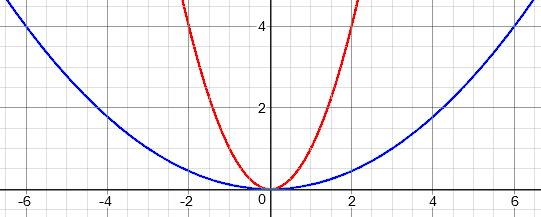The general formula for a quadratic function makes that decision for us, going with the vertical. If there were a horizontal translation, it would simply be the location of the vertex, with no adjustment needed for the stretching.

Next, try the same algebraic work I did above with an absolute value function, to see a similar effect.

Both functions x^2 and |x| have the property that

f(ab) = f(a)f(b)

This is the key that makes it possible to pull the multiplier outside. As a result, we don't NEED horizontal dilations (which many people consider the trickiest of transformations), because they don't give us anything we can't do without them. (You can still, of course, be given a quadratic function including a horizontal dilation, and need to be able to graph it.)

The same is true of various other functions, such as 1/x; but it is NOT true of any trig functions. Can you see why a periodic function couldn't have the necessary property? It's not periodicity directly ...

Thanks for a fun question.

One term for this property is that they are “multiplicative functions”; you might think of it as distributing over multiplication. This is true of any power, not only $$x^2$$ and $$x^{1/2} = \sqrt{x}$$ and $$x^{-1} = \frac{1}{x}$$.

## Reflection and symmetry

I have one more interesting interaction. This question is from 2017, and covers some of what we discussed last time, together with a new issue:

Order of Transformations of a Function, Yet Again

In school, we learned about absolute value translations, but we never covered what would happen if y = |-x|. In that particular equation, nothing changes.

But what if y = |-x + 1|?

I have found that y = |-x + 1| translates the graph to the right instead of the left.

And what happens if y = |-2x + 1|?

None of this is covered in school.

There are really two issues here. I replied:

The issues you are noticing are not really related to the absolute value so much as to combining two horizontal transformations.

As you note, y = |-x| looks the same as y = |x|. This is because the absolute value function has symmetry about the y-axis, so that reflection over the y-axis has no effect.

For your specific case, note that

|-x + 1| = |-(x - 1)|
= |x - 1|

As you say, this is translated to the right rather than to the left.

Here, as I have done before, I rewrote the shift-first form to the stretch-first form, which in this case is reflect-first. So we first reflect the graph about the y-axis, which has no effect due to symmetry, and then shift.

But this is a special case of something you need to know for all functions.

In general, for ANY function f, if you don't take the reflection into account, f(-x + 1) is translated "the wrong way."

The symmetry of the absolute value hides the reflection, which means you don't see what's really happening. So let's focus on the general case.

The rest is a recap of the general principles I discussed last time, with a little more going on than in any example there:

I like to think of these transformations step by step. And when we are working with the horizontal transformations (translate and stretch horizontally, and reflect over the y-axis), we have to be careful about order, which, like everything else, works backwards.

You have to think of each transformation as REPLACING the variable with an expression:

f(x)               original graph
f(x + 1)           translated left 1
f(-x + 1)          reflected over y-axis, which moves it
from left to RIGHT!

The reflection step replaces x with -x, so it has to be done last to get the function we want. To make things more natural (because our minds naturally tend to see it this way), we can do the reflection first, which will result in a different form for the function:

f(x)               original graph
f(-x)              reflected over y-axis
f(-(x - 1))        translated RIGHT 1

Do you see how this is similar to what I did with the absolute value function?

y = |-2x + 1|

Here is a sequence of transformations to obtain it:

y = |x|            original graph
y = |x + 1|        translated left 1
y = |-x + 1|       reflected over y-axis (DOES have an effect)
y = |-2x + 1|      shrunk by factor of 1/2 horizontally

If you carry these out carefully in this order, you will get the right graph (which you can verify by checking a few points).

But it is easy to get it wrong. You can instead rewrite it in factored form as

y = |x|            original graph
y = |-x|           reflected over y-axis (no effect)
y = |-2x|          shrunk by factor of 1/2 horizontally
y = |-2(x - 1/2)|  translated RIGHT 1/2

Again, this is the same as y = |2(x - 1/2)| with no reflection.

Now we’re ready for a bigger problem that I will look at next time.

This site uses Akismet to reduce spam. Learn how your comment data is processed.